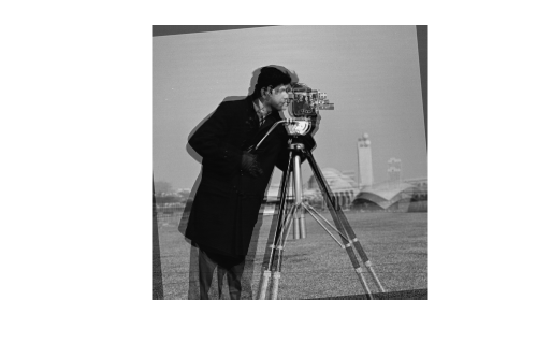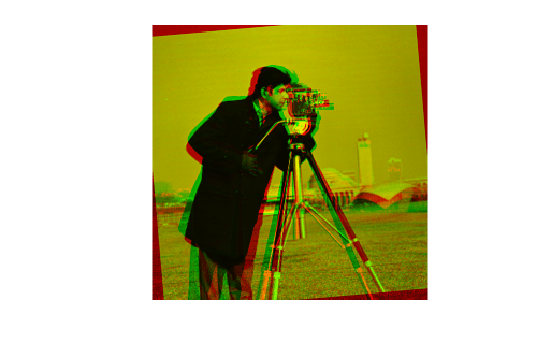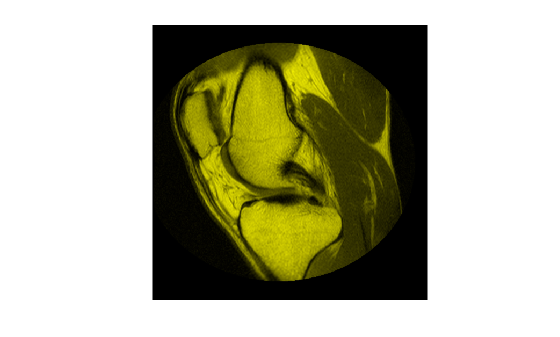# imfuse

Composite of two images

## Syntax

``C = imfuse(A,B)``
``````[C RC] = imfuse(A,RA,B,RB)``````
``C = imfuse(___,method)``
``C = imfuse(___,Name,Value)``

## Description

example

````C = imfuse(A,B)` creates a composite image from two images, `A` and `B`. If `A` and `B` are different sizes, `imfuse` pads the smaller dimensions with zeros so that both images are the same size before creating the composite. The output, `C`, is a numeric matrix containing a fused version of images `A` and `B`.```

example

``````[C RC] = imfuse(A,RA,B,RB)``` creates a composite image from two images, `A` and `B`, using the spatial referencing information provided in `RA` and `RB`. The output `RC` defines the spatial referencing information for the output fused image `C`.```

example

````C = imfuse(___,method)` uses the algorithm specified by `method`.```

example

````C = imfuse(___,Name,Value)` specifies additional options with one or more `Name,Value` pair arguments, using any of the previous syntaxes.```

## Examples

collapse all

Load an image into the workspace. Create a copy with a rotation offset applied.

```A = imread('cameraman.tif'); B = imrotate(A,5,'bicubic','crop');```

Create blended overlay image, scaling the intensities of `A` and `B` jointly as a single data set. View the fused image.

```C = imfuse(A,B,'blend','Scaling','joint'); imshow(C)```Save the resulting image as a `.png` file.

`imwrite(C,'my_blend_overlay.png');`

Load an image into the workspace. Create a copy and apply a rotation offset.

```A = imread('cameraman.tif'); B = imrotate(A,5,'bicubic','crop');```

Create a blended overlay image, using red for image `A`, green for image `B`, and yellow for areas of similar intensity between the two images. Then, display the overlay image.

```C = imfuse(A,B,'falsecolor','Scaling','joint','ColorChannels',[1 2 0]); imshow(C)```Save the resulting image as a `.png` file.

`imwrite(C,'my_blend_red-green.png');`

Load an image into the workspace and create a spatial referencing object associated with it.

```A = dicomread('knee1.dcm'); RA = imref2d(size(A));```

Create a second image by resizing image `A` and create a spatial referencing object associated with that image.

```B = imresize(A,2); RB = imref2d(size(B));```

Set referencing object parameters to specify the limits of the coordinates in world coordinates.

```RB.XWorldLimits = RA.XWorldLimits; RB.YWorldLimits = RA.YWorldLimits;```

Create a blended overlay image using color to indicate areas of similar intensity. This example uses red for image `A`, green for image `B`, and yellow for areas of similar intensity between the two images.

`C = imfuse(A,B,'falsecolor','Scaling','joint','ColorChannels',[1 2 0]);`

Display the fused image. Note how the images do not appear to share many areas of similar intensity. For this example, the fused image is shrunk for easier display.

```C = imresize(C,0.5); imshow(C)```Create a new fused image, this time using the spatial referencing information in `RA` and `RB`.

`[D,RD] = imfuse(A,RA,B,RB,'ColorChannels',[1 2 0]);`

Display the new fused image. In this version, the image appears yellow because the images `A` and `B` have the same extent in the world coordinate system. The images actually are aligned, even though `B` is twice the size of `A`. For this example, the fused image is shrunk for easier display.

```D = imresize(D,0.5); imshow(D)```## Input Arguments

collapse all

Image to be combined into a composite image, specified as a grayscale, truecolor, or binary image.

Data Types: `single` | `double` | `int8` | `int16` | `int32` | `int64` | `uint8` | `uint16` | `uint32` | `uint64` | `logical`

Image to be combined into a composite image, specified as a grayscale, truecolor, or binary image.

Data Types: `single` | `double` | `int8` | `int16` | `int32` | `int64` | `uint8` | `uint16` | `uint32` | `uint64` | `logical`

Spatial referencing information associated with the input image `A`, specified as a spatial referencing object of class `imref2d`.

Spatial referencing information associated with the input image `B`, specified as a spatial referencing object of class `imref2d`.

Algorithm used to combine images, specified as one of the following values.

MethodDescription
`'falsecolor'`Creates a composite RGB image showing `A` and `B` overlaid in different color bands. Gray regions in the composite image show where the two images have the same intensities. Magenta and green regions show where the intensities are different. This is the default method.
`'blend'`Overlays `A` and `B` using alpha blending.
`'checkerboard'`Creates an image with alternating rectangular regions from `A` and `B`.
`'diff'`Creates a difference image from `A` and `B`.
`'montage'`Puts `A` and `B` next to each other in the same image.

### Name-Value Pair Arguments

Specify optional comma-separated pairs of `Name,Value` arguments. `Name` is the argument name and `Value` is the corresponding value. `Name` must appear inside quotes. You can specify several name and value pair arguments in any order as `Name1,Value1,...,NameN,ValueN`.

Example: `'Scaling','joint'` scales the intensity values of `A` and `B` together as a single data set.

Intensity scaling option, specified as one of the following values:

 `'independent'` Scales the intensity values of `A` and `B` independently when `C` is created. `'joint'` Scales the intensity values in the images jointly as if they were together in the same image. This option is useful when you want to visualize registrations of monomodal images, where one image contains fill values that are outside the dynamic range of the other image. `'none'` No additional scaling.

Output color channel for each input image, specified as one of the following values:

 `[R G B]` A three element vector that specifies which image to assign to the red, green, and blue channels. The R, G, and B values must be 1 (for the first input image), 2 (for the second input image), and 0 (for neither image). `'red-cyan'` A shortcut for the vector `[1 2 2]`, which is suitable for red/cyan stereo anaglyphs `'green-magenta'` A shortcut for the vector `[2 1 2]`, which is a high contrast option, ideal for people with many kinds of color blindness

## Output Arguments

collapse all

Fused image that is a composite of the input images, returned as a grayscale, truecolor, or binary image.

Data Types: `uint8`

Spatial referencing information, returned as a spatial referencing object.

## Tips

• Use `imfuse` to create composite visualizations that you can save to a file. Use `imshowpair` to display composite visualizations to the screen.